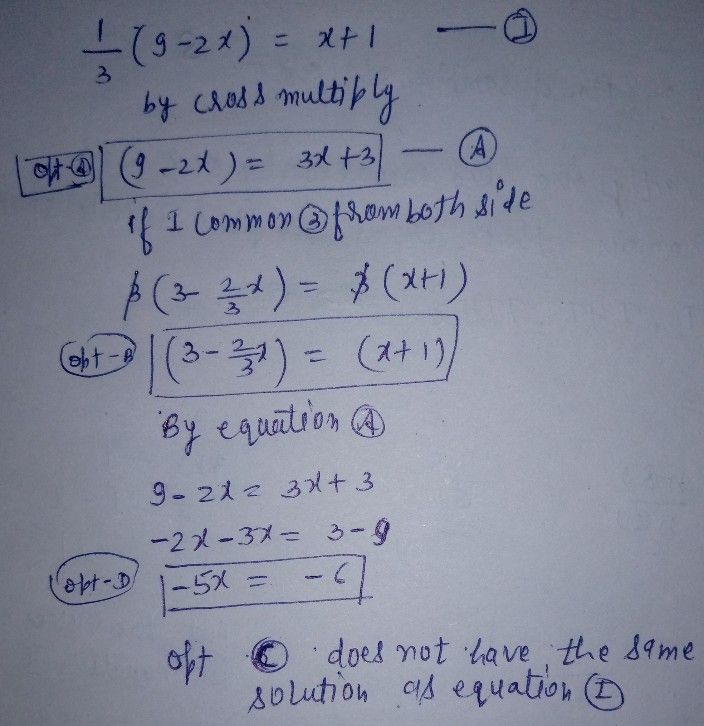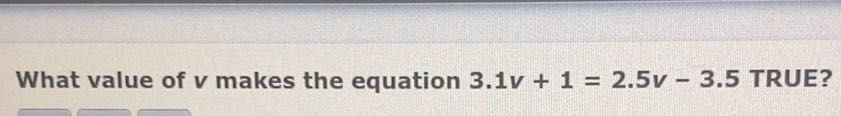Symbol
ProblemWhich equation does NOT have the same solution as $s\dfrac {1} {3}\left(9-2x\right)=x+12$ $\square$ $A$ A. $9-2x=3x+3$ $\square$ $B$ B. $3-\dfrac {2} {3}x=x+1$ $\square$ $C$ $3-2x=x+1$ $\square$ $\square$ D. $-5x=-6$